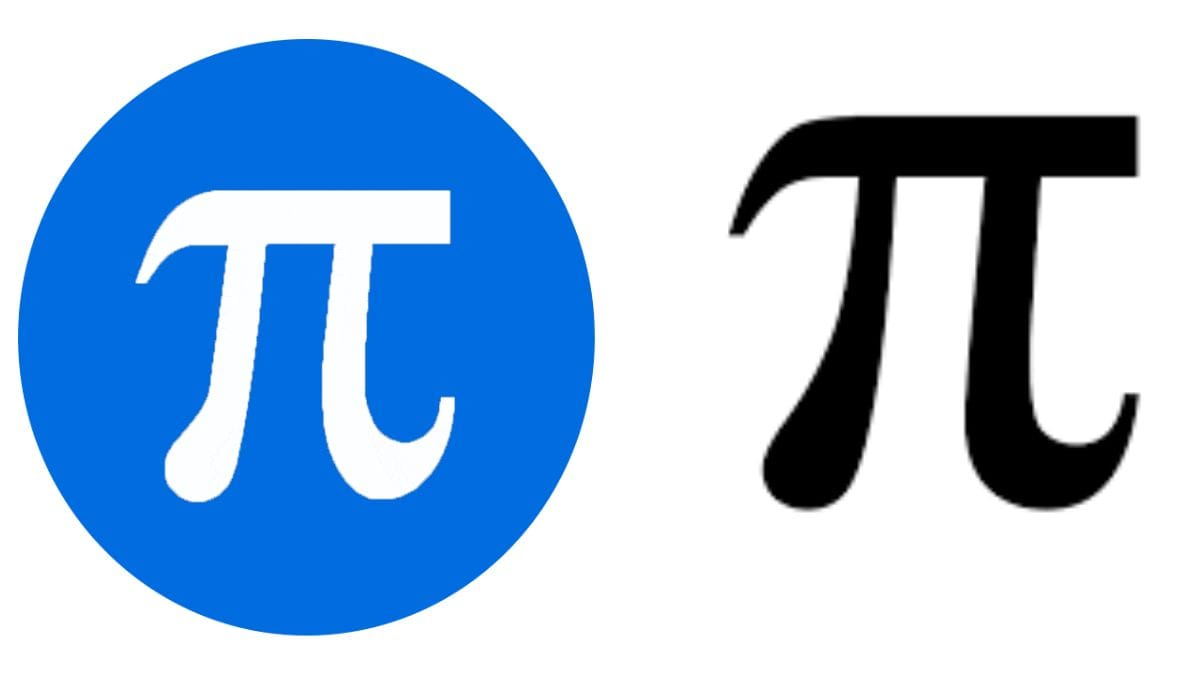Pi (π ) Approximation Day Observed On July 22; Know Reason Here

# Pi (π ) Approximation Day Observed On July 22; Know Reason Here

## The number π is a mathematical constant that is the ratio of circle's circumference to its diameter, approximately equal to 3.14159. A number of people celebrate March 14 as Pi Day as the date lines up in the numbers of the famous constant 3.14. However, such a format of writing dates is mostly followed in the US.

FPJ Education DeskUpdated: Friday, July 21, 2023, 08:05 PM ISTπ approximation Day |

Every year July 22 is observed as Pi Approximation Day due to this interesting reason. According to the date format, DD/MM, July 22 can be written as 22/7 which is the same value as approximate value of π, accurate to two decimal places and dates from Archimedes.

History of Pi Approximation Day

Pi (π) was known and used ancient times approximated for almost 4000 years. The ancient Babylonians also calculated the area of the circle by taking three times the square of its radius which gave the value of pi equals to three. Archimedes of Syracuse (287–212 BC), one of the greatest mathematicians of the ancient world was the first person to do the calculation of π. Archimedes tried to show that the value π is between 3 1/7 and 3 10/71.

Pi Approximation day is a observed annually to celebrate the usage of the mathematical constant π (pi). Pie day is observed on March 14 and Pi Approximation Day is observed on July 22. Pie day was founded by Larry Shaw in the year 1988. On November 2019 United Nations designated Pi day as the International Day of Mathematics.

What is Pi?

Pi is the ratio of the circumference of any circle to the diameter of that circle. It is represented as the Greek letter for p or Pi (π). Regardless of the size of the circle, the ratio is always equal to Pi, which is fractionally represented as 22/7 or 3.14 in the decimal form.

According to the book, A History of Pi by Petr Beckmann, the Greek letter π was first used by William Jones in 1706. It is believed Jones used the letter as an abbreviation of the periphery, which turned into a standard mathematical notation nearly 30 years later.

In 1737, Leonhard Euler adopted and popularised the symbol of Pi. Georges Buffon, an 18th-century French mathematician, invented a way to calculate Pi based on probability.